Weber Number Calculators Enter value, select unit and click on calculate. Result will be displayed.

Calculate Weber Number
Calculate Surface Tension
Calculate Density
Calculate Velocity
Calculate Length

 Web www.calculatoredge.com

Calculate Weber Number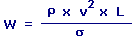W = Weber Number r = Density v = Velocity L = Length s = Surface Tension
 Enter your values: Density: Kilogram / Meter^3 Gram / Centimeter^3 Pound / Foot^3 Gram / Meter^3 Milligram / Liter Velocity: Meter / Second Foot / Second Centimeter / Hour Centimeter / Second Foot / Day Inch / Hour Inch / Second Kilometer / Second Knot Meter / Day Mile / Hour Mile / Second Millimeter / Second Yard / Second Length: Meter Centimeter Attometer Dekameter Decimeter Exameter Femtometer Foot Hectometer Inch Kilometer Megameter Micrometer Mile Millimeter Nanometer Petameter Picometer Yard Surface Tension: Newton / Meter Pound / Foot Pound / Inch Result: Weber Number:

Calculate Surface Tension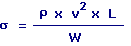s = Surface Tension r = Density v = Velocity L = Length W = Weber Number
 Enter your values: Density: Kilogram / Meter^3 Gram / Centimeter^3 Pound / Foot^3 Gram / Meter^3 Milligram / Liter Velocity: Meter / Second Foot / Second Centimeter / Hour Centimeter / Second Foot / Day Inch / Hour Inch / Second Kilometer / Second Knot Meter / Day Mile / Hour Mile / Second Millimeter / Second Yard / Second Length: Meter Centimeter Attometer Dekameter Decimeter Exameter Femtometer Foot Hectometer Inch Kilometer Megameter Micrometer Mile Millimeter Nanometer Petameter Picometer Yard Weber Number: Result: Surface Tension: Newton/Meter

Calculate Density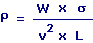r = Density s = Surface Tension W = Weber Number v = Velocity L = Length
 Enter your values: Weber Number: Surface Tension: Newton / Meter Pound / Foot Pound / Inch Velocity: Meter / Second Foot / Second Centimeter / Hour Centimeter / Second Foot / Day Inch / Hour Inch / Second Kilometer / Second Knot Meter / Day Mile / Hour Mile / Second Millimeter / Second Yard / Second Length: Meter Centimeter Attometer Dekameter Decimeter Exameter Femtometer Foot Hectometer Inch Kilometer Megameter Micrometer Mile Millimeter Nanometer Petameter Picometer Yard Result: Density: Kilogram/Meter3

Calculate Velocity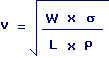v = Velocity W = Weber Number r = Density L = Lengths = Surface Tension
 Enter your values: Weber Number: Surface Tension: Newton / Meter Pound / Foot Pound / Inch Density: Kilogram / Meter^3 Gram / Centimeter^3 Pound / Foot^3 Gram / Meter^3 Milligram / Liter Length: Meter Centimeter Attometer Dekameter Decimeter Exameter Femtometer Foot Hectometer Inch Kilometer Megameter Micrometer Mile Millimeter Nanometer Petameter Picometer Yard Result: Velocity: Meter/Second

Calculate Length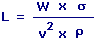L = Length W = Weber Number r = Densityv = Velocity s = Surface Tension
 Enter your values: Weber Number: Surface Tension: Newton / Meter Pound / Foot Pound / Inch Density: Kilogram / Meter^3 Gram / Centimeter^3 Pound / Foot^3 Gram / Meter^3 Milligram / Liter Velocity: Meter / Second Foot / Second Centimeter / Hour Centimeter / Second Foot / Day Inch / Hour Inch / Second Kilometer / Second Knot Meter / Day Mile / Hour Mile / Second Millimeter / Second Yard / Second Result: Length: MeterLINKSDISCLAIMERCONTACT US